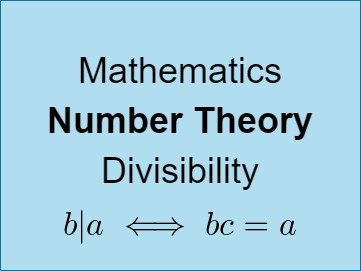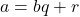# Mathematics - Number Theory - Divisibility

in STEMGeekslast year (edited)## Introduction

Hey it's a me again @drifter1!

Today we continue with Mathematics, and more specifically the "Number Theory" series, in order to get into Divisibility. It's a very important concept, which needs to be covered before we can get into more complicated ideas.

So, without further ado, let's get straight into it!

## Divisibility

Let's consider any two natural numbers a and b. Dividing these two numbers gives us a quotient q and remainder r. For example, dividing any a by b can be written as:If the remainder r is zero or otherwise the result of division is a so called "whole" number, then b divides a exactly, or a is said to be divisible by b.

For example, dividing 8 by 2 yields 4, and so 8 is divisible by 2 or 2 divides 8.

Mathematically, we commonly denote the "divides" word by a vertical line "|" and so "2 divides 8" becomes "2 | 8".

So, let's now use this notation to specify the concept of divisibility.

For any two natural numbers a and b we say that b | a if there exists an natural c so that bc = a, where c is the "whole" number result (or quotient) of the division: a ÷ b with no remainder.

Note: The concept of divisibility also applies to integers. For example, it's allowed to write "2 | -8" as 2 divides -8. But, we will be covering mostly positive integers (or naturals) during the series.

## Divisibility Rules

Numbers are so fascinating that there exist some very basic rules which can be used in order to check if a specific number is divisible by another:

### 1-rule

Of course any natural number is divisible by 1 and itself.

Zero is divisible by any number other then itself.

### 2-rule

Any number with 0, 2, 4, 6, or 8 as last digit is divisible by 2.

• 56 = 28 × 2 (divisible by 2).
• 57 = 28 × 2 + 1 (not divisible by 2).

### 3-rule

Any number with sum of digits divisible by 3 is also divisible by 3.

• 4131 = 1377 × 3, as 4 + 1 + 3 + 1 = 9, which is divisible by 3

### 4-rule

Any number where the last two digits are divisible by 4 is also divisible by 4.

• 380 = 95 × 4 as 80 is divisible by 4.

### 5-rule

Any number with 0 or 5 as last digit is divisible by 5.

• 55 = 11 × 5 (divisible by 5).
• 63 = 12 × 5 + 3 (not divisible by 5).

### 6-rule

Any even (divisible by 2) number which is divisible by 3 is also divisible by 6 (both 2-rule and 3-rule).

• 246 = 41 × 6, as 2 + 4 + 6 = 12 which is divisible by 3 and number is even.

### 7-rule

If doubling the last digit and substracting it from the number made by the other digits of a number is divisible by 7 then the number is also divisible by 7.

• 238 = 34 × 7, as 23 - (2 × 8) = 23 - 16 = 7 which is divisible by 7.

### 8-rule

Any number is divisble by 8 if its last three digits are divisible by 8.

• 36536 = 4567 × 8 as 536 = 67 × 8 and so divisible by 8.

### 9-rule

Any number with sum of digits divisible by 9 is also divisible by 9.

• 516132 = 57348 × 9 as 5 + 1 + 6 + 1 + 3 + 2 = 18 which is divisible by 9.

### 10-rule

Any number which ends with 0 is divisible by 10.

• 137583183810 (divisible by 10).

### 11-rule

If the result of adding and subtracting digits in alternating pattern (add-subtract-add-subtract) is divisible by 11 than the number is divisible by 11.

• 8294 = 754 × 11 as 8 - 2 + 9 - 4 = 11 which is divisible by 11.

### 12-rule

Any number which is divisible by both 3 and 4 is also divisible by 12.

• 408 = 34 × 12 as 4 + 8 = 12 which is divisible by 3 and "08" is divisible by 4.

Exercise:

Try to check if 5280 is divisible by 2-to-12 by applying these rules. Have fun!

## RESOURCES:

### References

Block diagrams and other visualizations were made using draw.io.

## Previous articles of the series

• Introduction → Number Theory, Why Number Theory, Series Outline

## Final words | Next up

And this is actually it for today's post!

Next time we will cover the Euclidean Division Algorithm, which was already in this article as an Easter egg. Can you spot it?

See ya!Keep on drifting!

Posted with STEMGeeks

Sort:
last year

That rule for 7 is definitely something I didn't know before.

!discovery 31

Yeah I remember those cheats in division from school lol
!1UP

PIZZA!

PIZZA Holders sent \$PIZZA tips in this post's comments:
@curation-cartel(16/20) tipped @drifter1 (x1)#### You have received a 1UP from @gwajnberg!

The @oneup-cartel will soon upvote you with:
@stem-curator
And they will bring !PIZZA 🍕.This post was shared and voted inside the discord by the curators team of discovery-it
Discovery-it is also a Witness, vote for us here
Delegate to us for passive income. Check our 80% fee-back Program

Your content has been voted as a part of Encouragement program. Keep up the good work!

Use Ecency daily to boost your growth on platform!

Support Ecency
Vote for new Proposal
Delegate HP and earn more

last year (edited)

Nice one drifter. Now I am thinking to bring how to make divisibility rule for any number.😃😃

Drifting of a car is pretty awesome.haha

last year Reveal Comment